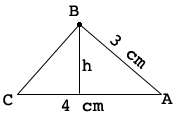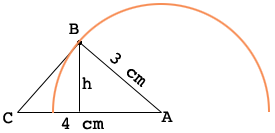SEARCH HOMEMath Central Quandaries & QueriesQuestion from Tom: Triangle ABC is such that AB=3cm and AC=4cm. What is the maximum possible area of triangle ABC? Thank you for your time.Hi Tom,

Here is a diagram of one such triangle.It's area is

$\frac12 \times 4 \times h \mbox{ square centimeters.}$

In my second diagram the orange curve is an arc of a circle with center $A$ and radius 3 cm.The point $B$ must be on this arc if the length of $AB$ is 3 cm. What point on the arc makes $h$ a maximum and hence gives a triangle of maximum area?

PennyMath Central is supported by the University of Regina and The Pacific Institute for the Mathematical Sciences.